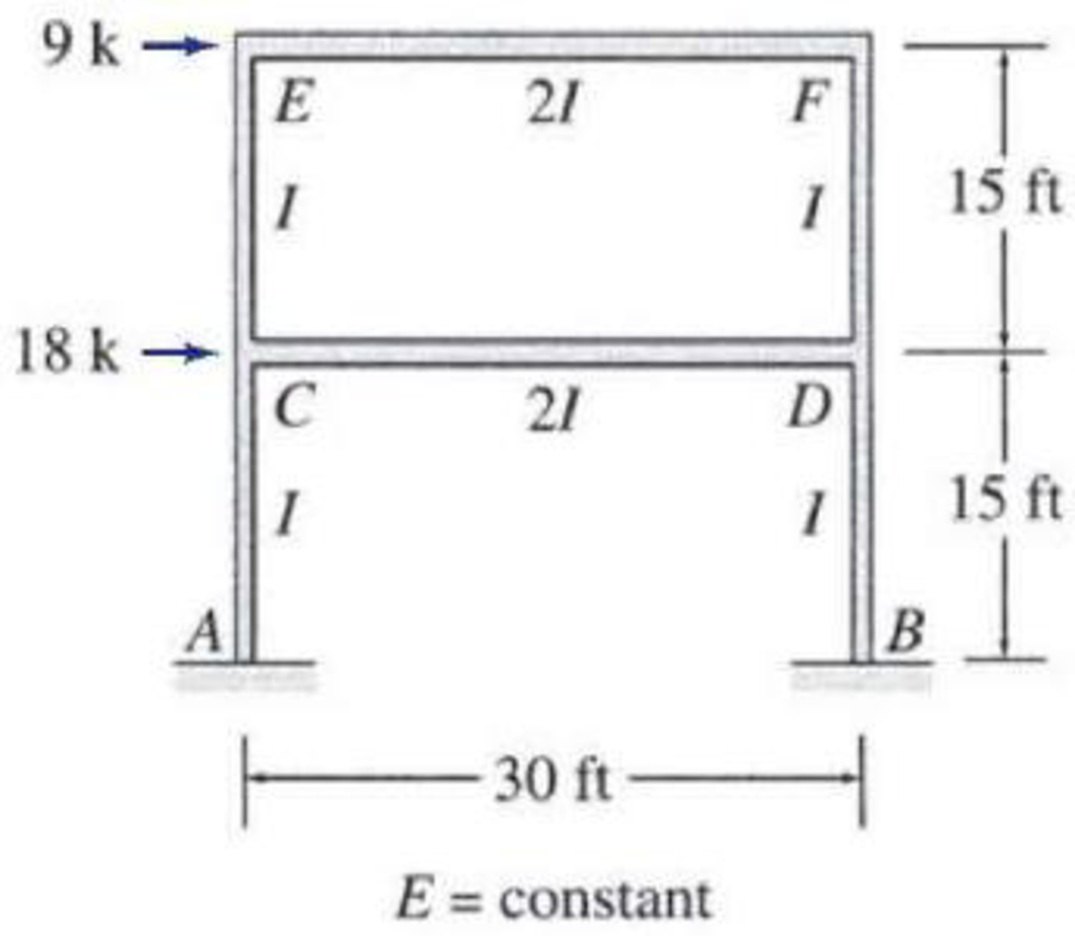# 15.24 through 15.31 Determine the member end moments and reactions for the frames shown in Figs. P15.24–P15.31 by using the slope-deflection method. FIG. P15.31

#### Solutions

Chapter
Section
Chapter 15, Problem 31P
Textbook Problem
41 views

## 15.24 through 15.31 Determine the member end moments and reactions for the frames shown in Figs. P15.24–P15.31 by using the slope-deflection method.FIG. P15.31To determine

Find the member end moments and reaction for the frames.

### Explanation of Solution

Calculation:

Consider the elastic modulus E of the frame is constant.

Show the free body diagram of the entire frame as in Figure 1.

Refer Figure 1,

Calculate the fixed end moment for AC.

FEMAC=0

Calculate the fixed end moment for CA.

FEMCA=0

Calculate the fixed end moment for CD.

FEMCD=0

Calculate the fixed end moment for DC.

FEMDC=0

Calculate the fixed end moment for DB.

FEMDB=0

Calculate the fixed end moment for BD.

FEMBD=0

Calculate the fixed end moment for CE.

FEMCE=0

Calculate the fixed end moment for EC.

FEMEC=0

Calculate the fixed end moment for EF.

FEMEF=0

Calculate the fixed end moment for FE.

FEMFE=0

Calculate the fixed end moment for FD.

FEMFD=0

Calculate the fixed end moment for DF.

FEMDF=0

Chord rotations:

Show the free body diagram of the chord rotation of the frame as in Figure 2.

Refer Figure 2,

Calculate the chord rotation of the frame AC and BD.

ψAC=ψBD=Δ115

Calculate the chord rotation of the frame CE and DF.

ψCE=ψDF=Δ215

Calculate the chord rotation of the frame CD and EF.

ψCD=ψEF=0

Calculate the slope deflection equation for the member AC.

MAC=2EIL(2θA+θC3ψAC)+FEMAC

Substitute 15 ft for L, 0 for θA, Δ115 for ψAC, and 0 for FEMAC.

MAC=2EI15(2(0)+θC3(Δ115))+0=0.133EIθC+0.0267EIΔ1        (1)

Calculate the slope deflection equation for the member CA.

MCA=2EIL(2θC+θA3ψCA)+FEMCA

Substitute 15 ft for L, 0 for θA, Δ115 for ψCA, and 0 for FEMCA.

MCA=2EI15(2θC+03(Δ115))0=0.2667EIθC+0.0267EIΔ1        (2)

Calculate the slope deflection equation for the member CD.

MCD=2E(2I)L(2θC+θDψCD)+FEMCD

Substitute 30 ft for L, 0 for ψCD, and 0 for FEMCD.

MCD=2E(2I)30(2θC+θD3(0))+0=0.2667EIθC+0.133EIθD        (3)

Calculate the slope deflection equation for the member DC.

MDC=2E(2I)L(2θD+θCψDC)+FEMDC

Substitute 30 ft for L, 0 for ψDC, and 0 for FEMDC.

MDC=2E(2I)30(2θD+θC3(0))0=0.133EIθC+0.2667EIθD        (4)

Calculate the slope deflection equation for the member DB.

MDB=2EIL(2θD+θB3ψDB)+FEMDB

Substitute 15 ft for L, 0 for θB, Δ115 for ψDB, and 0kft for FEMDB.

MDB=2EI15(2θD+(0)3(Δ115))+0=0.2667EIθD+0.0267EIΔ1        (5)

Calculate the slope deflection equation for the member BD.

MBD=2EIL(2θB+θD3ψBD)+FEMBD

Substitute 15 ft for L, 0 for θB, Δ115 for ψBD, and 0kft for FEMBD.

MBD=2EI15(θD+2(0)3(Δ115))+0=0.133EIθD+0.0267EIΔ1        (6)

Calculate the slope deflection equation for the member CE.

MCE=2EIL(2θC+θE3ψCE)+FEMCE

Substitute 15 ft for L, Δ215 for ψCE, and 0 for FEMCE.

MCE=2EI15(2θC+θE3(Δ215))+0=0.2667EIθC+0.133EIθE+0.0267EIΔ2        (7)

Calculate the slope deflection equation for the member EC.

MEC=2EIL(2θE+θC3ψEC)+FEMEC

Substitute 15 ft for L, Δ215 for ψEC and 0 for FEMEC.

MEC=2EI15(2θE+θC3(Δ215))+0=0.133EIθC+0.2667EIθE+0.0267EIΔ2        (8)

Calculate the slope deflection equation for the member EF.

MEF=2E(2I)L(2θE+θFψEF)+FEMEF

Substitute 30 ft for L, 0 for ψEF and 0 for FEMEF.

MEF=2E(2I)30(2θE+θF3(0))+0=0.2667EIθE+0.133EIθF        (9)

Calculate the slope deflection equation for the member FE.

MFE=2E(2I)L(2θF+θEψFE)+FEMFE

Substitute 30 ft for L, 0 for ψFE and 0 for FEMFE.

MFE=2E(2I)30(2θF+θE3(0))+0=0.133EIθE+0.2667EIθF        (10)

Calculate the slope deflection equation for the member FD.

MFD=2EIL(2θF+θD3ψFD)+FEMFD

Substitute 15 ft for L, Δ215 for ψFD and 0kft for FEMFD.

MFD=2EI15(2θF+θD3(Δ215))+0=0.2667EIθF+0.133EIθD+0.0267EIΔ2        (11)

Calculate the slope deflection equation for the member DF.

MDF=2EIL(2θD+θF3ψDF)+FEMDF

Substitute 15 ft for L, Δ215 for ψDF, and 0kft for FEMDF.

MDF=2EI15(2θD+θF3(Δ215))+0=0.2667EIθD+0.133EIθF+0.0267EIΔ2        (12)

Write the equilibrium equation as below.

MCA+MCD+MCE=0

Substitute equation (2), equation (3), and equation (7) in above equation.

{0.2667EIθC+0.0267EIΔ1+0.2667EIθC+0.133EIθD+0.2667EIθC+0.133EIθE+0.0267EIΔ2}=00.8EIθC+0.133EIθD+0.133EIθE+0.0267EIΔ1+0.0267EIΔ2=0        (13)

Write the equilibrium equation as below.

MDC+MDB+MDF=0

Substitute equation (4), equation (5) and equation (12) in above equation.

{0.133EIθC+0.2667EIθD+0.2667EIθD+0.0267EIΔ1+0.2667EIθD+0.133EIθF+0.0267EIΔ2}=00.133EIθC+0.8EIθD++0.133EIθF+0.0267EIΔ1+0.0267EIΔ2=0        (14)

Write the equilibrium equation as below.

MEC+MEF=0

Substitute equation (8) and equation (9) in above equation.

{0.133EIθC+0.2667EIθE+0.0267EIΔ2+0.2667EIθE+0.133EIθF}=00.133EIθC+0.533EIθE+0.133EIθF+0.0267EIΔ2=0        (15)

Write the equilibrium equation as below.

MFD+MFE=0

Substitute equation (10) and equation (11) in above equation.

{0.133EIθE+0.2667EIθF+0.2667EIθF+0.133EIθD+0.0267EIΔ2}=00.133EIθE+0.533EIθF+0.133EIθD+0.0267EIΔ2=0        (16)

Show the free body diagram of the joint E and F due to sway force as in Figure 3.

Calculate the horizontal reaction at the member CE due to sway force by taking moment about point C.

MC=0(SCE×15)+MCE+MEC=0SCE=MCE+MEC15

Calculate the horizontal reaction at the member DF due to sway force by taking moment about point D.

MD=0(SDF×15)+MDF+MFD=0SDF=MDF+MFD15

Calculate the reaction of the support E and support F due to sway force by considering horizontal equilibrium.

+Fx=0SCE+SDF=9(MCE+MEC15)+(MDF+MFD15)=9MCE+MEC+MDF+MFD15=9MCE+MEC+MDF+MFD=135

Substitute equation (7), (8), (11) and (12)

### Still sussing out bartleby?

Check out a sample textbook solution.

See a sample solution

#### The Solution to Your Study Problems

Bartleby provides explanations to thousands of textbook problems written by our experts, many with advanced degrees!

Get Started

Find more solutions based on key concepts
As explained in earlier chapters, fins, or extended surfaces, commonly are used in a variety of engineering app...

Engineering Fundamentals: An Introduction to Engineering (MindTap Course List)

Determine the resultant of the line load acting on the beam.

International Edition---engineering Mechanics: Statics, 4th Edition

Identify tools word processing programs provide that can assist you when writing.

Enhanced Discovering Computers 2017 (Shelly Cashman Series) (MindTap Course List)

Name three items that are manufactured using welding.

Welding: Principles and Applications (MindTap Course List)

If your motherboard supports ECC DDR3 memory, can you substitute non-ECC DDR3 memory?

A+ Guide to Hardware (Standalone Book) (MindTap Course List)